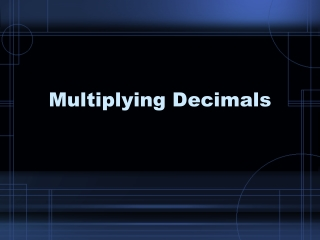Download PresentationMultiplying Decimals

# Multiplying Decimals

Télécharger la présentation## Multiplying Decimals

- - - - - - - - - - - - - - - - - - - - - - - - - - - E N D - - - - - - - - - - - - - - - - - - - - - - - - - - -
##### Presentation Transcript

1. Multiplying Decimals

2. To Multiply: • You do not line up the factors by the decimal. • Instead, place the number with more digits on top. • Line up the other number underneath, at the right. • Multiply • Count the number of decimal places (from the right) in each factor. • Use the total number of decimal places in your two factors to place the decimal in your product.

3. Example: 5.63 x 3.7 1 4 2 5.63 two x 3.7 one 1 1 39 4 1 1 + 16 8 9 0 2 0 . 8 3 1 three

4. Example: 0.53 x 2.618 2.618 has more digits (4) than 0.53 (3), so it goes on top. 3 4 Decimal Places 1 2 2.618 three x 0.53 two 1 7 8 5 4 13 0 9 0 0 + 0 0 0 0 00 . 1 3 8 7 5 4 five

5. Try This: 6.5 x 15.3 3 1 2 1 15.3 one x 6.5 one 1 7 6 5 + 9 1 8 0 . 9 9 4 5 two

6. Multiplication Properties Commutative Property of Multiplication: Factors can be multiplied in any order. Example: 12 x 5 = 5 x 12 Associative Property of Multiplication: Factors can be grouped in any way. Example: (2 x 3) x 5 = 2 x (3 x 5) Identity Property of Multiplication: The product of any number and one is that number. Example: 18 x 1 = 18